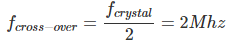# MCQs on Microprocessor

##### Page 5 of 19. Go to page 1 2 3 4 5 6 7 8 9 10 11 12 13 14 15 16 17 18 19
01․ The first machine cycle of an instruction is always
A fetch cycle
A memory write cycle

The first machine cycle of an instruction is always fetch cycle.

02․ The output data lines of microprocessor and memories are usually tristated because
More than one device can transmit information over the data bus by enabling only one device at a time
More than one device can transmit over the data bus at the same time
The data line can be multiplexed for both input and output
It increases the speed of data transfer over the data bus

Using tristate buffer, more than one device can transmit information over the data bus by enabling only one device at a time.

03․ The correct sequence of steps in the instruction cycle of a basic computer is

At first instruction is fetched then decoded and then read effective address and executed.

04․ Following is a 16-bit register for 8085 microprocessor
Stack pointer
Accumulator
Register
Register C

In a 8085 microprocessor Accumulator, register B & C are of 8-bit whereas stack pointer is a 16-bit register.

05․ The register which holds the information about the nature of results of arithmetic and logic operations is called as
Accumulator
Condition code register
Flag register
Process status register

In a microprocessor flag registers indicate status of an arithmetic and logic operation.

06․ When referring to instruction words, a mnemonic is
a short abbreviation for the operand address.
a short abbreviation for the operation to be performed.
a short abbreviation for the data word stored at the operand address.
shorthand for machine language.

All instructions consist of opcode and operand where opcode indicates operation to be performed and operand is on which operation to be done. Opcode is always expressed as a short abbreviation which is nothing but mnemonic.

07․ While using a frequency counter for measuring frequency, two modes of measurement are possible:
1. Period time
2. Frequency mode
There is a ‘cross-over frequency’ below which the period mode is preferred. Assuming the crystal oscillator frequency to be 4MHz, the crossover frequency is given by
8 Mhz.
2 Mhz.
2 Khz.
1Khz.

As we know crossover frequency is the half of oscillator frequency. i.e.08․ In 8085 microprocessor system with memory mapped I/O, which of the following is true?
Devices are accessed using IN and OUT instructions
There can be maximum of 256 input devices and 256 output devices
Arithmetic and logic operations can be directly performed with the I/O data

With the memory mapped I/O arithmetic and logic operations can be directly performed whereas with I/O mapped I/O it is not possible. Options A, B, C are valid for I/O mapped I/O.

09․ Consider the following statements: Arithmetic Logic Unit (ALU) 1.Performs arithmetic operations 2.Performs comparisons. 3.Communicates with I/O devices 4.Keeps watch on the system Which of these statements are correct?
1, 2, 3 and 4
1, 2 and 3
1 and 2 only
3 and 4 only

ALU performs arithmetic and logical operations. Note: Comparison is a logical operation.

10․ Ready pin of microprocessor is used